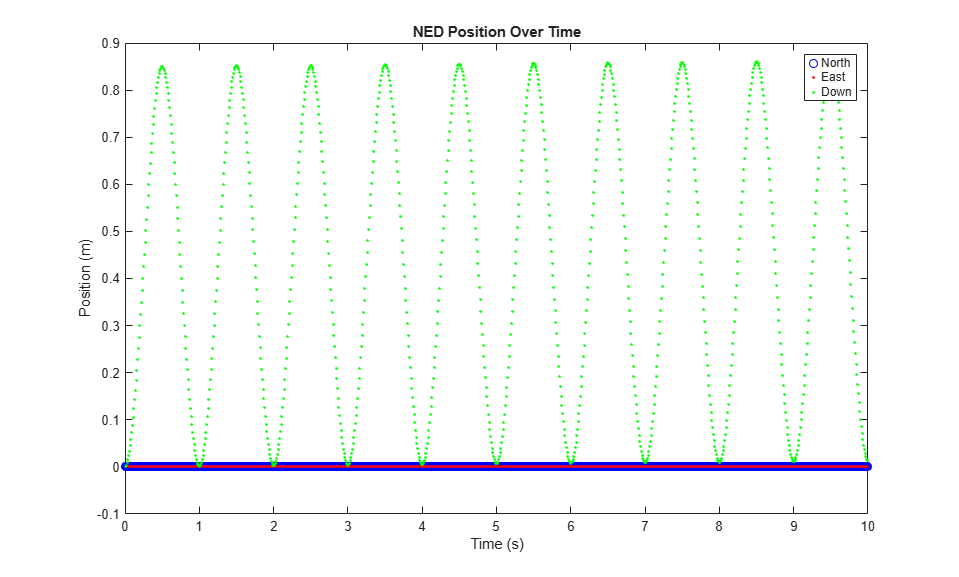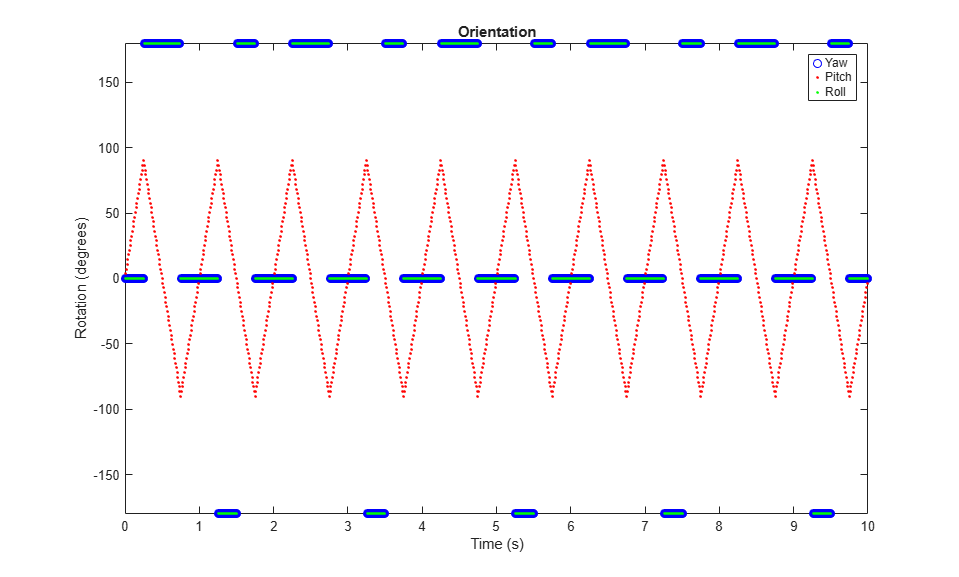kinematicTrajectory

Rate-driven trajectory generator

Description

The kinematicTrajectory System object™ generates trajectories using specified acceleration and angular velocity.

To generate a trajectory from rates:

1. Create the kinematicTrajectory object and set its properties.

2. Call the object with arguments, as if it were a function.

Creation

Description

example

trajectory = kinematicTrajectory returns a System object, trajectory, that generates a trajectory based on acceleration and angular velocity.

example

trajectory = kinematicTrajectory(Name,Value) sets each property Name to the specified Value. Unspecified properties have default values.

Example: trajectory = kinematicTrajectory('SampleRate',200,'Position',[0,1,10]) creates a kinematic trajectory System object, trajectory, with a sample rate of 200 Hz and the initial position set to [0,1,10].

Properties

expand all

If a property is tunable, you can change its value at any time.

Sample rate of trajectory in Hz, specified as a positive scalar.

Tunable: Yes

Data Types: single | double

Position state in the local navigation coordinate system in meters, specified as a three-element row vector.

Tunable: Yes

Data Types: single | double

Velocity state in the local navigation coordinate system in m/s, specified as a three-element row vector.

Tunable: Yes

Data Types: single | double

Orientation state in the local navigation coordinate system, specified as a scalar quaternion or 3-by-3 real matrix. The orientation is a frame rotation from the local navigation coordinate system to the current body frame.

Tunable: Yes

Data Types: quaternion | single | double

Source of acceleration state, specified as 'Input' or 'Property'.

• 'Input' –– specify acceleration state as an input argument to the kinematic trajectory object

• 'Property' –– specify acceleration state by setting the Acceleration property

Tunable: No

Data Types: char | string

Acceleration state in m/s2, specified as a three-element row vector.

Tunable: Yes

Dependencies

To enable this property, set AccelerationSource to 'Property'.

Data Types: single | double

Source of angular velocity state, specified as 'Input' or 'Property'.

• 'Input' –– specify angular velocity state as an input argument to the kinematic trajectory object

• 'Property' –– specify angular velocity state by setting the AngularVelocity property

Tunable: No

Data Types: char | string

Angular velocity state in rad/s, specified as a three-element row vector.

Tunable: Yes

Dependencies

To enable this property, set AngularVelocitySource to 'Property'.

Data Types: single | double

Number of samples per output frame, specified as a positive integer.

Tunable: No

Dependencies

To enable this property, set AngularVelocitySource to 'Property' and AccelerationSource to 'Property'.

Data Types: single | double

Usage

Description

example

[position,orientation,velocity,acceleration,angularVelocity] = trajectory(bodyAcceleration,bodyAngularVelocity) outputs the trajectory state and then updates the trajectory state based on bodyAcceleration and bodyAngularVelocity.

This syntax is only valid if AngularVelocitySource is set to 'Input' and AccelerationSource is set to 'Input'.

[position,orientation,velocity,acceleration,angularVelocity] = trajectory(bodyAngularVelocity) outputs the trajectory state and then updates the trajectory state based on bodyAngularAcceleration.

This syntax is only valid if AngularVelocitySource is set to 'Input' and AccelerationSource is set to 'Property'.

[position,orientation,velocity,acceleration,angularVelocity] = trajectory(bodyAcceleration) outputs the trajectory state and then updates the trajectory state based on bodyAcceleration.

This syntax is only valid if AngularVelocitySource is set to 'Property' and AccelerationSource is set to 'Input'.

[position,orientation,velocity,acceleration,angularVelocity] = trajectory() outputs the trajectory state and then updates the trajectory state.

This syntax is only valid if AngularVelocitySource is set to 'Property' and AccelerationSource is set to 'Property'.

Input Arguments

expand all

Acceleration in the body coordinate system in meters per second squared, specified as an N-by-3 matrix.

N is the number of samples in the current frame.

Angular velocity in the body coordinate system in radians per second, specified as an N-by-3 matrix.

N is the number of samples in the current frame.

Output Arguments

expand all

Position in the local navigation coordinate system in meters, returned as an N-by-3 matrix.

N is the number of samples in the current frame.

Data Types: single | double

Orientation in the local navigation coordinate system, returned as an N-by-1 quaternion column vector or a 3-by-3-by-N real array. Each quaternion or 3-by-3 rotation matrix is a frame rotation from the local navigation coordinate system to the current body coordinate system.

N is the number of samples in the current frame.

Data Types: single | double

Velocity in the local navigation coordinate system in meters per second, returned as an N-by-3 matrix.

N is the number of samples in the current frame.

Data Types: single | double

Acceleration in the local navigation coordinate system in meters per second squared, returned as an N-by-3 matrix.

N is the number of samples in the current frame.

Data Types: single | double

Angular velocity in the local navigation coordinate system in radians per second, returned as an N-by-3 matrix.

N is the number of samples in the current frame.

Data Types: single | double

Object Functions

expand all

 perturbations Perturbation defined on object perturb Apply perturbations to object
 step Run System object algorithm

Examples

collapse all

Create a default kinematicTrajectory System object™ and explore the relationship between input, properties, and the generated trajectories.

trajectory = kinematicTrajectory
trajectory =
kinematicTrajectory with properties:

SampleRate: 100
Position: [0 0 0]
Orientation: [1x1 quaternion]
Velocity: [0 0 0]
AccelerationSource: 'Input'
AngularVelocitySource: 'Input'

By default, the kinematicTrajectory object has an initial position of [0 0 0] and an initial velocity of [0 0 0]. Orientation is described by a quaternion one (1 + 0i + 0j + 0k).

The kinematicTrajectory object maintains a visible and writable state in the properties Position, Velocity, and Orientation. When you call the object, the state is output and then updated.

For example, call the object by specifying an acceleration and angular velocity relative to the body coordinate system.

bodyAcceleration = [5,5,0];
bodyAngularVelocity = [0,0,1];
[position,orientation,velocity,acceleration,angularVelocity] = trajectory(bodyAcceleration,bodyAngularVelocity)
position = 1×3

0     0     0

orientation = quaternion
1 + 0i + 0j + 0k

velocity = 1×3

0     0     0

acceleration = 1×3

5     5     0

angularVelocity = 1×3

0     0     1

The position, orientation, and velocity output from the trajectory object correspond to the state reported by the properties before calling the object. The trajectory state is updated after being called and is observable from the properties:

trajectory
trajectory =
kinematicTrajectory with properties:

SampleRate: 100
Position: [2.5000e-04 2.5000e-04 0]
Orientation: [1x1 quaternion]
Velocity: [0.0500 0.0500 0]
AccelerationSource: 'Input'
AngularVelocitySource: 'Input'

The acceleration and angularVelocity output from the trajectory object correspond to the bodyAcceleration and bodyAngularVelocity, except that they are returned in the navigation coordinate system. Use the orientation output to rotate acceleration and angularVelocity to the body coordinate system and verify they are approximately equivalent to bodyAcceleration and bodyAngularVelocity.

rotatedAcceleration = rotatepoint(orientation,acceleration)
rotatedAcceleration = 1×3

5     5     0

rotatedAngularVelocity = rotatepoint(orientation,angularVelocity)
rotatedAngularVelocity = 1×3

0     0     1

The kinematicTrajectory System object™ enables you to modify the trajectory state through the properties. Set the position to [0,0,0] and then call the object with a specified acceleration and angular velocity in the body coordinate system. For illustrative purposes, clone the trajectory object before modifying the Position property. Call both objects and observe that the positions diverge.

trajectoryClone = clone(trajectory);
trajectory.Position = [0,0,0];

position = trajectory(bodyAcceleration,bodyAngularVelocity)
position = 1×3

0     0     0

clonePosition = trajectoryClone(bodyAcceleration,bodyAngularVelocity)
clonePosition = 1×3
10-3 ×

0.2500    0.2500         0

This example shows how to create a trajectory oscillating along the North axis of a local NED coordinate system using the kinematicTrajectory System object™.

Create a default kinematicTrajectory object. The default initial orientation is aligned with the local NED coordinate system.

traj = kinematicTrajectory
traj =

kinematicTrajectory with properties:

SampleRate: 100
Position: [0 0 0]
Orientation: [1x1 quaternion]
Velocity: [0 0 0]
AccelerationSource: 'Input'
AngularVelocitySource: 'Input'

Define a trajectory for a duration of 10 seconds consisting of rotation around the East axis (pitch) and an oscillation along North axis of the local NED coordinate system. Use the default kinematicTrajectory sample rate.

fs = traj.SampleRate;
duration = 10;

numSamples = duration*fs;

cyclesPerSecond = 1;
samplesPerCycle = fs/cyclesPerSecond;
numCycles = ceil(numSamples/samplesPerCycle);
maxAccel = 20;

triangle = [linspace(maxAccel,1/fs-maxAccel,samplesPerCycle/2), ...
linspace(-maxAccel,maxAccel-(1/fs),samplesPerCycle/2)]';
oscillation = repmat(triangle,numCycles,1);
oscillation = oscillation(1:numSamples);

accNED = [zeros(numSamples,2),oscillation];

angVelNED = zeros(numSamples,3);
angVelNED(:,2) = 2*pi;

Plot the acceleration control signal.

timeVector = 0:1/fs:(duration-1/fs);

figure(1)
plot(timeVector,oscillation)
xlabel('Time (s)')
ylabel('Acceleration (m/s)^2')
title('Acceleration in Local NED Coordinate System')Generate the trajectory sample-by-sample in a loop. The kinematicTrajectory System object assumes the acceleration and angular velocity inputs are in the local sensor body coordinate system. Rotate the acceleration and angular velocity control signals from the NED coordinate system to the sensor body coordinate system using rotateframe and the Orientation state. Update a 3-D plot of the position at each time. Add pause to mimic real-time processing. Once the loop is complete, plot the position over time. Rotating the accNED and angVelNED control signals to the local body coordinate system assures the motion stays along the Down axis.

figure(2)
plotHandle = plot3(traj.Position(1),traj.Position(2),traj.Position(3),'bo');
grid on
xlabel('North')
ylabel('East')
zlabel('Down')
axis([-1 1 -1 1 0 1.5])
hold on

q = ones(numSamples,1,'quaternion');
for ii = 1:numSamples
accBody = rotateframe(traj.Orientation,accNED(ii,:));
angVelBody = rotateframe(traj.Orientation,angVelNED(ii,:));

[pos(ii,:),q(ii),vel,ac] = traj(accBody,angVelBody);

set(plotHandle,'XData',pos(ii,1),'YData',pos(ii,2),'ZData',pos(ii,3))

pause(1/fs)
end

figure(3)
plot(timeVector,pos(:,1),'bo',...
timeVector,pos(:,2),'r.',...
timeVector,pos(:,3),'g.')
xlabel('Time (s)')
ylabel('Position (m)')
title('NED Position Over Time')
legend('North','East','Down')Convert the recorded orientation to Euler angles and plot. Although the orientation of the platform changed over time, the acceleration always acted along the North axis.

figure(4)
eulerAngles = eulerd(q,'ZYX','frame');
plot(timeVector,eulerAngles(:,1),'bo',...
timeVector,eulerAngles(:,2),'r.',...
timeVector,eulerAngles(:,3),'g.')
axis([0,duration,-180,180])
legend('Yaw','Pitch','Roll')
xlabel('Time (s)')
ylabel('Rotation (degrees)')
title('Orientation')This example shows how to generate a coil trajectory using the kinematicTrajectory System object™.

Create a circular trajectory for a 1000 second duration and a sample rate of 10 Hz. Set the radius of the circle to 5000 meters and the speed to 80 meters per second. Set the climb rate to 100 meters per second and the pitch to 15 degrees. Specify the initial orientation as pointed in the direction of motion.

duration = 1000; % seconds
fs = 10;         % Hz
N = duration*fs; % number of samples

speed = 80;      % meters per second
climbRate = 50;  % meters per second
initialYaw = 90; % degrees
pitch = 15;      % degrees

initVel = [0, speed, climbRate];
initOrientation = quaternion([initialYaw,pitch,0],'eulerd','zyx','frame');

trajectory = kinematicTrajectory('SampleRate',fs, ...
'Velocity',initVel, ...
'Position',initPos, ...
'Orientation',initOrientation);

Specify a constant acceleration and angular velocity in the body coordinate system. Rotate the body frame to account for the pitch.

accBody = zeros(N,3);
accBody(:,3) = 0.2;

angVelBody = zeros(N,3);

pitchRotation = quaternion([0,pitch,0],'eulerd','zyx','frame');
angVelBody = rotateframe(pitchRotation,angVelBody);
accBody = rotateframe(pitchRotation,accBody);

Call trajectory with the specified acceleration and angular velocity in the body coordinate system. Plot the position, orientation, and speed over time.

[position, orientation, velocity] = trajectory(accBody,angVelBody);

eulerAngles = eulerd(orientation,'ZYX','frame');
speed = sqrt(sum(velocity.^2,2));

timeVector = (0:(N-1))/fs;

figure(1)
plot3(position(:,1),position(:,2),position(:,3))
xlabel('North (m)')
ylabel('East (m)')
zlabel('Down (m)')
title('Position')
grid onfigure(2)
plot(timeVector,eulerAngles(:,1),...
timeVector,eulerAngles(:,2),...
timeVector,eulerAngles(:,3))
axis([0,duration,-180,180])
legend('Yaw (Rotation Around Down)','Pitch (Rotation Around East)','Roll (Rotation Around North)')
xlabel('Time (s)')
ylabel('Rotation (degrees)')
title('Orientation')figure(3)
plot(timeVector,speed)
xlabel('Time (s)')
ylabel('Speed (m/s)')
title('Speed')Define a constant angular velocity and constant acceleration that describe a spiraling circular trajectory.

Fs = 100;
r = 10;
speed = 2.5;
initialYaw = 90;

initPos = [r 0 0];
initVel = [0 speed 0];
initOrient = quaternion([initialYaw 0 0], 'eulerd', 'ZYX', 'frame');

accBody = [0 speed^2/r 0.01];
angVelBody = [0 0 speed/r];

Create a kinematic trajectory object.

traj = kinematicTrajectory('SampleRate',Fs, ...
'Position',initPos, ...
'Velocity',initVel, ...
'Orientation',initOrient, ...
'AccelerationSource','Property', ...
'Acceleration',accBody, ...
'AngularVelocitySource','Property', ...
'AngularVelocity',angVelBody);

Call the kinematic trajectory object in a loop and log the position output. Plot the position over time.

N = 10000;
pos = zeros(N, 3);
for i = 1:N
pos(i,:) = traj();
end

plot3(pos(:,1), pos(:,2), pos(:,3))
title('Position')
xlabel('X (m)')
ylabel('Y (m)')
zlabel('Z (m)')